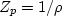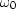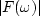Next: Two poles Up: LEAKY INTEGRATION Previous: LEAKY INTEGRATION

## Plots

A pole is a place in the complex plane where a filter B(Zp) becomes infinity. This occurs where a denominator vanishes. For example, in equation (8) we see that there is one pole and it is located at.In plots like Figure 1, a pole location is denoted by a p'' and a zero location by a z." I chose to display the pole and zero locations in the-plane instead of in the Z0-plane. Thus real frequencies run along the horizontal axis instead of around the circle of |Z|=1. I further chose to superpose the complex-plane on the graph ofversus.This enables us to correlate the pole and zero locations to the spectrum. I plottedin order that theandaxes would coincide. As we will see later, some poles give stable filters and some poles give unstable filters. At the risk of some confusion, I introduced the minus sign to put the stable poles atop the positive spectrum. Since we will never see a negative spectrum and we will rarely see an unstable pole, this economizes on paper (or maximizes resolution for a fixed amount of paper).

In Figure 1, moving the p'' down toward the horizontal axis would cause a slower time decay and a sharper frequency function.Next: Two poles Up: LEAKY INTEGRATION Previous: LEAKY INTEGRATION
Stanford Exploration Project
10/21/1998